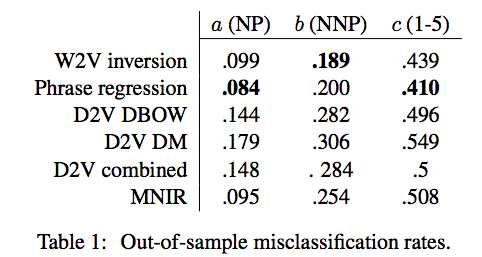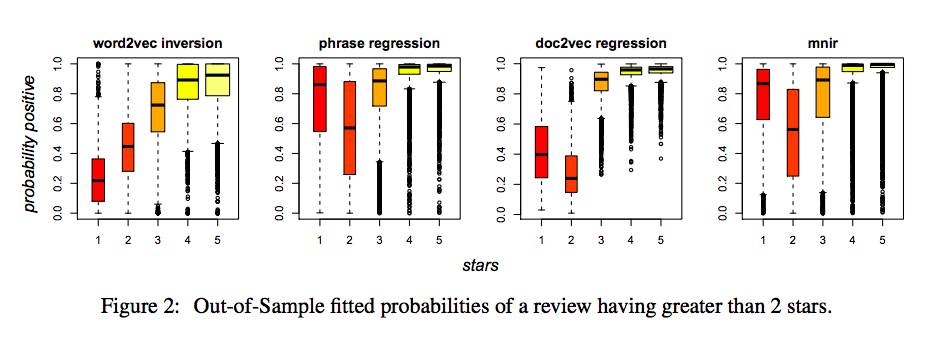# Document Classification by Inversion of Distributed Language Representations

This is a note on the arxiv by Matt Taddy from April 2015. It reads very clearly and has a simple point to make: language modelling techniques can be used in classification tasks by training a separate language model for each class; documents are assigned to the class of the model where the document has the highest likelihood (hence “inversion”). In our discussion, we assume a uniform prior over the classes.

Taddy considers the particular case of predicting the sentiment of Yelp reviews at different levels of granularity. Different approaches are considered:

• word2vec inversion is inversion in the sense described above where document vectors are taken as the average of the word vectors of the constituent words;
• phrase regression, where separate logistic regression models are trained for each output class, taking as input phrase count vectors;
• doc2vec regression, is as per phrase regression, but taking as input one of:
• doc2vec DBOW
• doc2vec DM
• doc2vec DBOW and DM combined, i.e. in direct sum
• MNIR, the authors own Multinomial Inverse Regression

Three separate classification tasks are considered, labelled “a”, “b” and “c” in the diagram below, representing two-, three- and five-class sentiment classification.As illustrated in the following figure, only the word2vec inversion technique would do a decent job when the gravity of a misclassification is considered (so penalising less if, e.g. predicted star rating is off by only one star):Missing from Taddy’s comparison is inversion using the document vectors, though this is certainly the sort of thing his paper suggests might work well. Also missing is regression using the document vectors obtained as aggregates of word vectors.